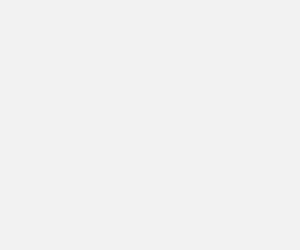# Logical Reasoning Dice Questions for CAT 2022 [Theoretical Concepts]CAT Exam Date: Nov 2022We need to be clear on fundamentals of Logical Reasoning to crack DILR Section in CAT 2022.

Time is opportune to be ready with LR to be successful in CAT 2022 which is being conducting in November 2022.

Logical Reasoning (LR) will be a test for your logical conclusions and you must not assume for answering questions in LR. Read following concepts:

Dice – Theoretical Concepts

Introduction:

Dice is a cube. In cube there are 6 faces. Some important points are given below:

1. There are 6 faces in the cube – ABCG,GCDE, DEFH, BCDH, AGEF and ABHF.2. Always four faces are adjacent to one face.
3. Opposite of ABCG is DEFH and so on.
4. CDEG is the upper faces of the cube.
5. ABHF is the bottom of the cube.

Rule No. 1:

Two opposite faces cannot be adjacent to one another.

Example:

Two different positions of the dice are shown below. Which number will appear on the face opposite to the face with number 4?Solution:

Faces with four number 6, 2, 5 and 3 are adjacent of to the face with No. 4.

Hence the faces with no. 6, 2, 5 and 3 cannot be opposite to the face with no. 4.

Therefore the remaining face with no.1 will be the opposite of the face with no. 4.

Rule No. 2:

If two different positions of a dice are shown and one of the two common faces is in the same position then of the remaining faces will be opposite to each other.

Example:

Two different position of a dice are shown below.Here in both shown positions two faces 5 and 3 are common.

The remaining faces are 2 and 4.

Hence the number on the face opposite to the face with number 2 is 4.

Rule No. 3

If in two different positions of dice, the position of a common face be the same, then each of the opposite faces of the remaining faces will be in the same position.

Example:Solution :

Here in both positions of common (3) is same.

Therefore, opposite of 5 is 6 and opposite of 4 is 2.

Rule No. 4

If in two different positions of a dice, the position of the common face be not the same, then opposite face of the common face will be that which is not shown on any face in these two positions. Besides, the opposite faces of the remaining faces will not be the same.

Example:Solution:

Here in two positions of a dice the face with number 1 is not in the same position.

The face with number 6 is not shown.

Hence the face opposite to the face with number 1 is 6.

Besides the opposite face of 3 will be the face with number 2 and opposite face to face 5 will be the face with number 1.

### Practice with  LR MOCKS

Stay informed, Stay ahead and stay inspired with MBA Rendezvous